# Consider the function f:R + R defined by if x is rational f(x) = if x...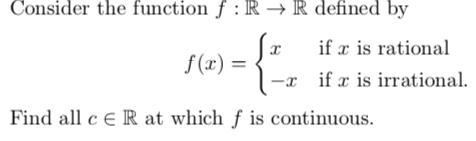Consider the function f:R + R defined by if x is rational f(x) = if x is irrational. Find all c € R at which f is continuous. C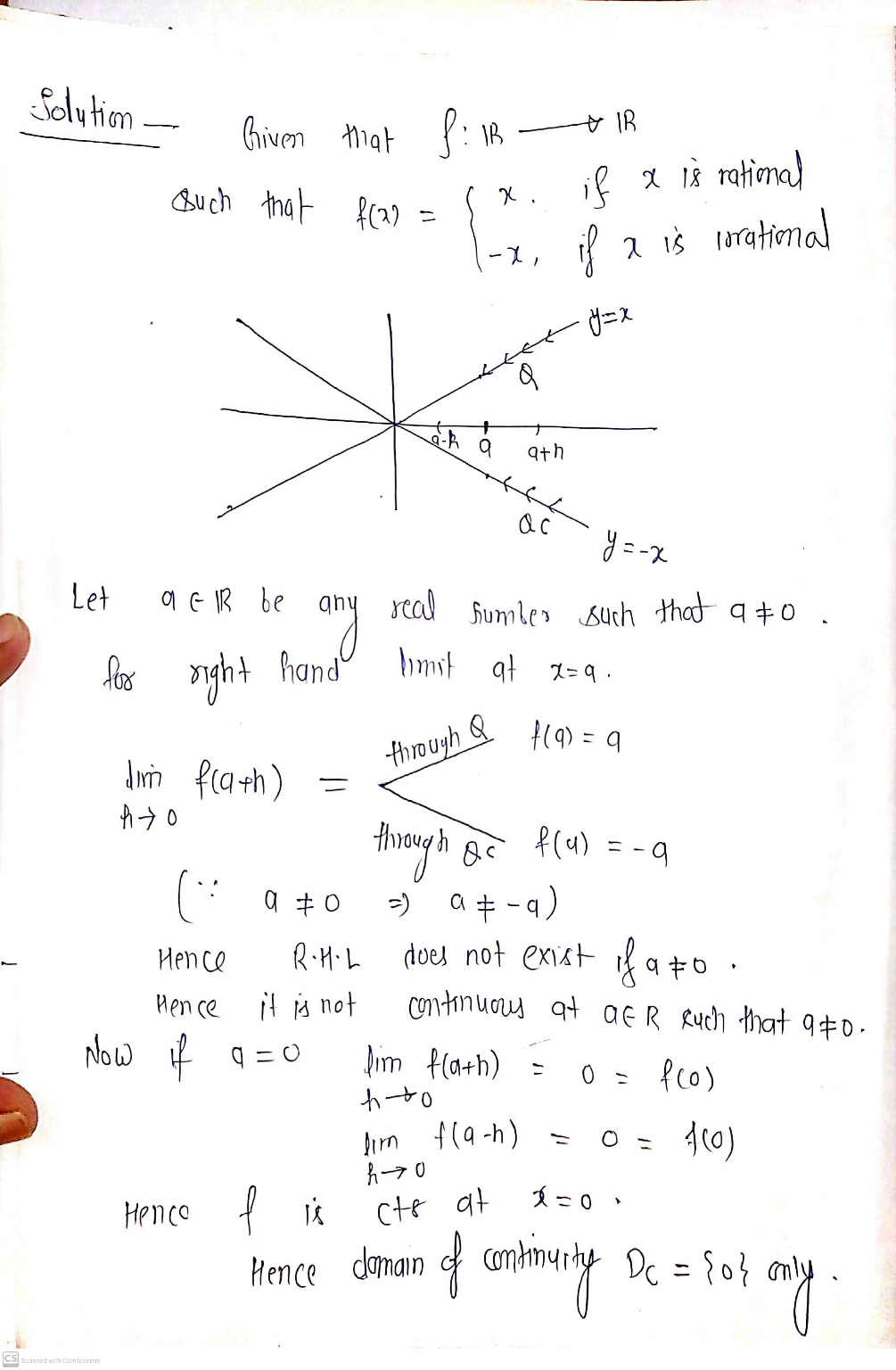##### Add Answer of: Consider the function f:R + R defined by if x is rational f(x) = if x...
Similar Homework Help Questions
• ### Consider the function f : R → R defined by f(x) = !x if x is...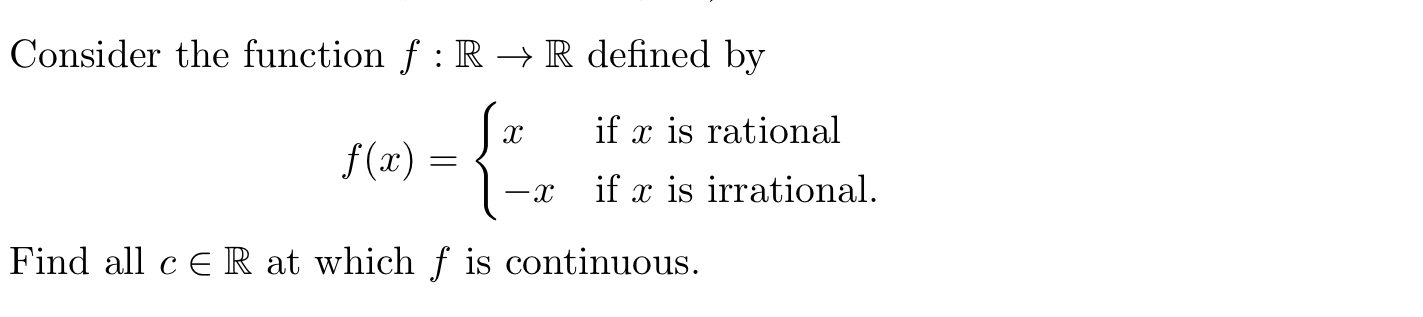Consider the function f : R → R defined by f(x) = !x if x is rational −x if x is irrational. Find all c ∈ R at which f is continuous. Consider the function f :R → R defined by .. х if x is rational f(x) = -2 if x is irrational. Find all c ER at which f is continuous.

• ### 1. Let f:R → R be the function defined as: 32 0 if x is rational...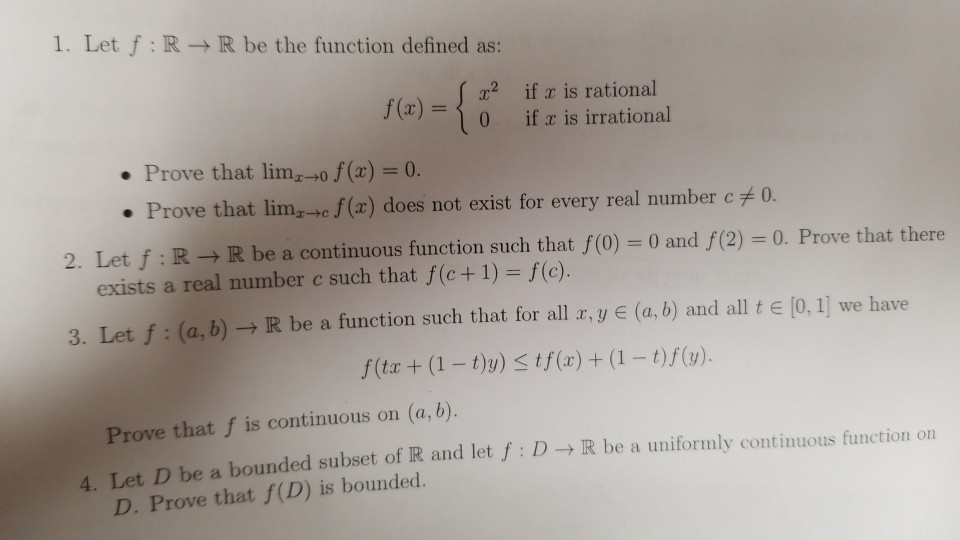1. Let f:R → R be the function defined as: 32 0 if x is rational if x is irrational Prove that lim -70 f(x) = 0. Prove that limc f(x) does not exist for every real number c + 0. 2. Let f:R + R be a continuous function such that f(0) = 0 and f(2) = 0. Prove that there exists a real number c such that f(c+1) = f(c). 3 Let f. (a,b) R be a function...

• ### (2) Consider the function f given by f:R R f(a)1 2 (a) Determine the domain D...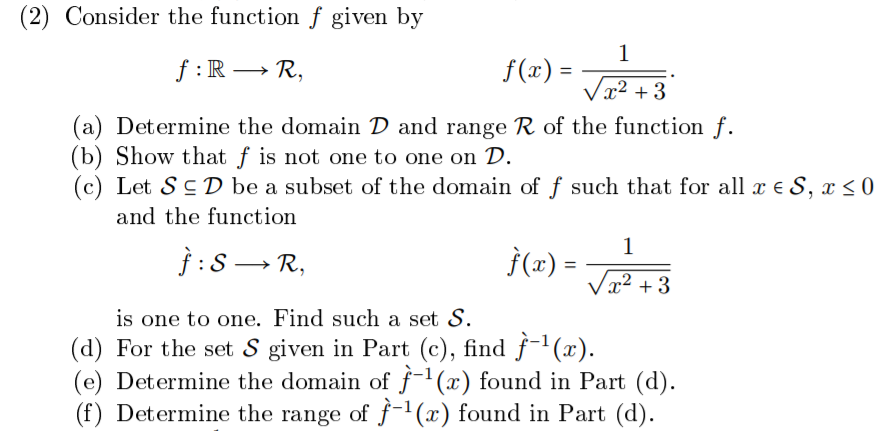(2) Consider the function f given by f:R R f(a)1 2 (a) Determine the domain D and range R of the function f. (b) Show that f is not one to one on D. (c) Let ç D be a subset of the domain of f such that for all x ? S, 0 and the function is one to one. Find such a set S. (d) For the set S given in Part (c), find f (x) (e) Determine...

• ### 1. Consider the function: f:R R defined by f()2z +5 cos(3z). (a) Compute the values f...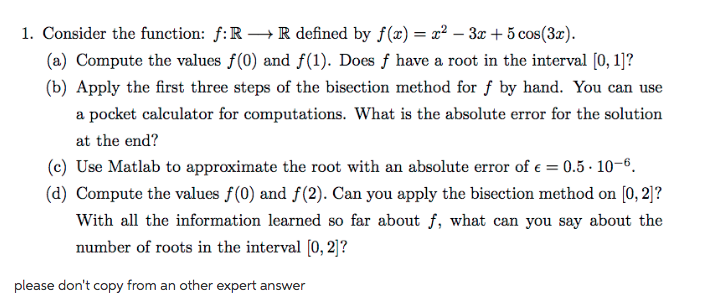1. Consider the function: f:R R defined by f()2z +5 cos(3z). (a) Compute the values f (0) and f(1). Does f have a root in the interval [0, 1]? (b) Apply the first three steps of the bisection method for f by hand. You can use a pocket calculator for computations. What is the absolute error for the solution at the end? (c) Use Matlab to approximate the root with an absolute error of e0.5-10-6. (d) Compute the values f(0)...

• ### Let a,b and c be real numbers and consider the function defined by . For which values of a,b,...

Let a,b and c be real numbers and consider the function defined by . For which values of a,b, and c is f one-to-one and or onto ? Show all work. f:R→R We were unable to transcribe this imageWe were unable to transcribe this image f:R→R

• ### points) Let f: R R be defined by setting f() = xif ris rational 8. (20...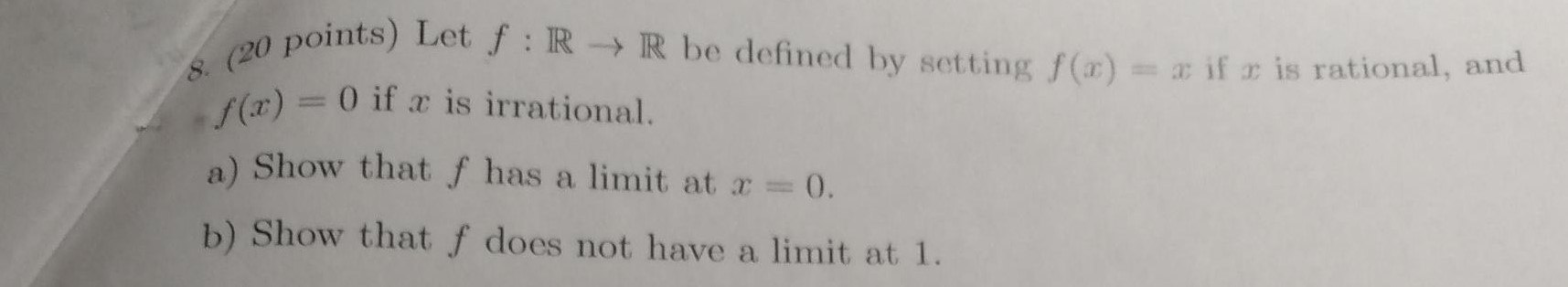points) Let f: R R be defined by setting f() = xif ris rational 8. (20 point (x) = 0 if x is irrational. a) Show that f has a limit at x = 0. b) Show that f does not have a limit at 1.

• ### real analysis II. Consider the function f:[0,1] - R defined by f(x) 0 if x E...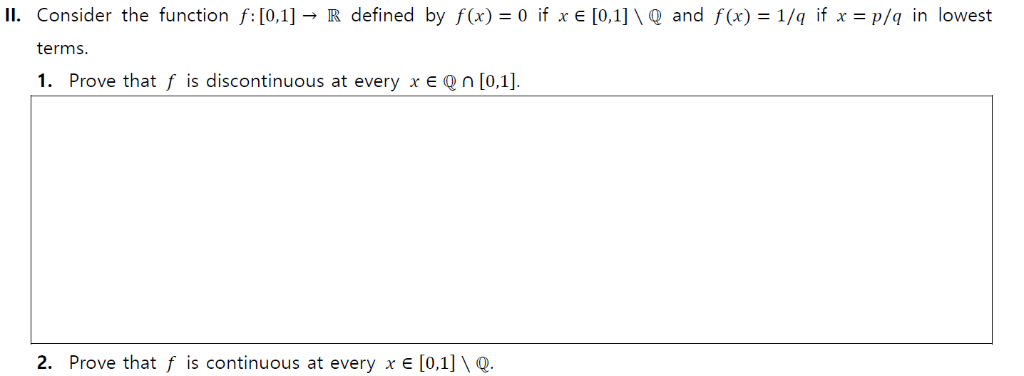real analysis II. Consider the function f:[0,1] - R defined by f(x) 0 if x E [0,1]\ Q and f(x) = 1/q if x = p/q in lowest terms. 1. Prove that f is discontinuous at every x E Qn [0,1]. 2. Prove that f is continuous at every x e [0,1] \ Q. II. Consider the function f:[0,1] - R defined by f(x) 0 if x E [0,1]\ Q and f(x) = 1/q if x = p/q in lowest...

• ### f:R->R is a differentiable function

f:R->R is a differentiable functionf(x+y)=f(x)+f(y)+xy(x+y)

• ### Consider the function f(x) = Σ (a) Where is f defined? (b) Where is f continuous? (c) Where is f ...

Consider the function f(x) = Σ (a) Where is f defined? (b) Where is f continuous? (c) Where is f differentiable? Consider the function f(x) = Σ (a) Where is f defined? (b) Where is f continuous? (c) Where is f differentiable?

• ### 2. Consider the function f : R2 → R defined below. r3уг_ if (x,y) (0,0) f(x,y)...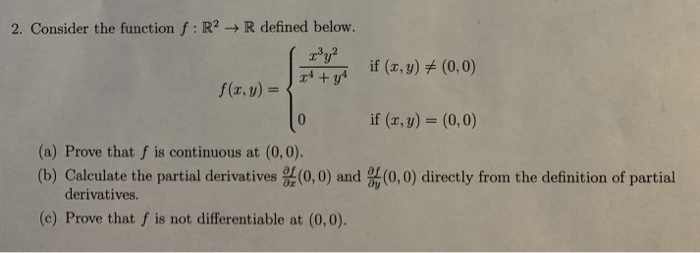2. Consider the function f : R2 → R defined below. r3уг_ if (x,y) (0,0) f(x,y) = if (x, y) (0, 0) (a) Prove that f is continuous at (0,0) (b) Calculate the partial derivatives (0,0) and (0,0) directly from the definition of partial derivatives. (c) Prove that f is not differentiable at (0,0).

Free Homework App